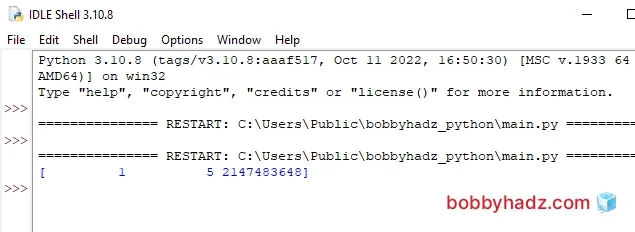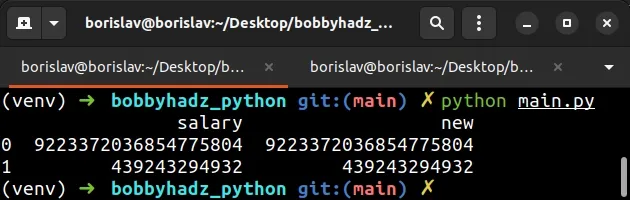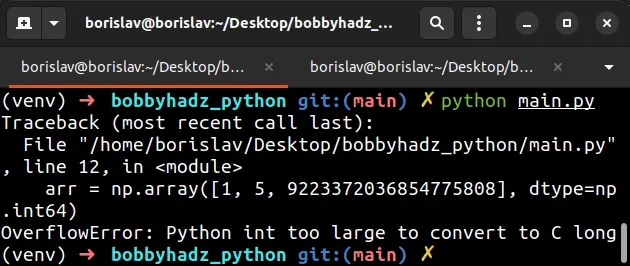# OverflowError: Python int too large to convert to C longLast updated: Jun 24, 2023
4 min## #OverflowError: Python int too large to convert to C long

The error "OverflowError: Python int too large to convert to C long" occurs when one or more of the supplied Python integers are too large to be converted to C long.

To solve the error, set the data type of the numbers to `np.int64` instead of `int`.

Here is an example of how the error occurs.

main.py
```Copied!```import numpy as np

# ⛔️ OverflowError: Python int too large to convert to C long
arr = np.array([1, 5, 2147483648], dtype=int)

print(arr)
``````One of the numbers we passed to the numpy.array method is too large to be converted to a C long.

• The `int32` (or `int`) data type can store integers from `-2147483648` to `2147483647`.
• On the other hand, the `int64` data type can store integers from `-9223372036854775808` to `9223372036854775807`.

To solve the error, set the `dtype` (data type) argument to `np.int64` instead.

main.py
```Copied!```import numpy as np

arr = np.array([1, 5, 2147483648], dtype=np.int64)

print(arr)
``````Note: you might still get the error if one of the numbers exceeds `sys.maxsize` (more on that below).

You can also set the `dtype` to `"int64"` to achieve the same result.

main.py
```Copied!```import numpy as np

arr = np.array([1, 5, 2147483648], dtype='int64')

print(arr)
``````

You won't get the error on macOS or Linux if the numbers are in the range from `-2147483648` to `2147483647`.

This is because the `int` (or `int32`) type uses a C `long` which is always 32-bit on Windows.

For example, the following code sample runs without any issues on macOS and Linux, but causes the error on Windows.

main.py
```Copied!```import numpy as np

arr = np.array([1, 5, 2147483648], dtype=int)

# [         1          5 2147483648]
print(arr)
``````On Windows, C `long` is 32-bit and on macOS and Linux, it is 64-bit.

## #Solving the error when using a pandas DataFrame

If you got the error when using a `pandas` DataFrame, use the `astype()` method to cast the pandas object to the `int64` `dtype`.

main.py
```Copied!```import pandas as pd

df = pd.DataFrame({'salary': ['9223372036854775804', '439243294932']})

df['new'] = df['salary'].astype('int64')

print(df)
``````The DataFrame.astype method takes a dtype (data type) as a parameter and casts the pandas object to the specified `dtype`.

## #The error is also raised when trying to store integers greater than `sys.maxsize`

The error is also raised when you try to store integers that are greater than sys.maxsize.

main.py
```Copied!```import sys

print(sys.maxsize) # 👉️ 9223372036854775807
``````The `sys.maxsize` property is an integer that defines the maximum value a variable of type Py_ssize_t can take.

On a 32-bit platform, the value is: `2**31 - 1` = `2147483647`.

On a 64-bit platform, the value is `2**63 - 1` = `9223372036854775807`.

If you try to store a value that is greater than `sys.maxsize` in a NumPy array, the error is raised.

main.py
```Copied!```import numpy as np

# ⛔️ OverflowError: Python int too large to convert to C long
arr = np.array([1, 5, 9223372036854775808], dtype=np.int64)
``````Note that native Python lists can store much larger integer values.

main.py
```Copied!```a_list = [1, 5, 92233720368547758088888888]

# [1, 5, 92233720368547758088888888]
print(a_list)
``````

If you have to store the values in a NumPy array, set the type to `np.float64` instead.

main.py
```Copied!```import numpy as np

arr = np.array([1, 5, 9223372036854775808], dtype=np.float64)

# [1.00000000e+00 5.00000000e+00 9.22337204e+18]
print(arr)
``````The `int` type uses a C `long` under the hood which is quite limited (especially on Windows).

You can use the `np.float64` data type to store larger values in a NumPy array.

You can also use the `float` type when working with a pandas `DataFrame`.

main.py
```Copied!```import pandas as pd

df = pd.DataFrame({'salary': ['9223372036854775808', '439243294932']})

df['new'] = df['salary'].astype(float)

print(df)
``````In general, NumPy arrays are not suited for storing extremely large integer values.

You can either use a native Python list or set the data type of the sequence to `float` or `np.float64`.

If you try to store an integer that is greater than `sys.maxsize`, you would get the "OverflowError: Python int too large to convert to C long" error.